# A Quick Introduction to iNEXT via Examples

#### 2020-01-28

iNEXT (iNterpolation and EXTrapolation) is an R package modified from the original version which was supplied in the Supplement of Chao et al. (2014). In the latest updated version, we have added more user‐friendly features and refined the graphic displays. In this document, we provide a quick introduction demonstrating how to run iNEXT. Detailed information about iNEXT functions is provided in the iNEXT Manual, also available in CRAN. See Chao & Jost (2012), Colwell et al. (2012) and Chao et al. (2014) for methodologies. A short review of the theoretical background and a brief description of methods are included in an application paper by Hsieh, Ma & Chao (2016). An online version of iNEXT (http://chao.stat.nthu.edu.tw/wordpress/software_download/inext-online/) is also available for users without an R background.

iNEXT focuses on three measures of Hill numbers of order q: species richness (q = 0), Shannon diversity (q = 1, the exponential of Shannon entropy) and Simpson diversity (q = 2, the inverse of Simpson concentration). For each diversity measure, iNEXT uses the observed sample of abundance or incidence data (called the “reference sample”) to compute diversity estimates and the associated 95% confidence intervals for the following two types of rarefaction and extrapolation (R/E):

1. Sample‐size‐based R/E sampling curves: iNEXT computes diversity estimates for rarefied and extrapolated samples up to an appropriate size. This type of sampling curve plots the diversity estimates with respect to sample size.
2. Coverage‐based R/E sampling curves: iNEXT computes diversity estimates for rarefied and extrapolated samples with sample completeness (as measured by sample coverage) up to an appropriate coverage. This type of sampling curve plots the diversity estimates with respect to sample coverage.

iNEXT also plots the above two types of sampling curves and a sample completeness curve. The sample completeness curve provides a bridge between these two types of curves.

## HOW TO RUN INEXT:

The iNEXT package is available on CRAN and can be downloaded with a standard R installation procedure using the following commands. For a first‐time installation, an additional visualization extension package (ggplot2) must be loaded.

## install iNEXT package from CRAN
install.packages("iNEXT")

install.packages('devtools')
library(devtools)
install_github('JohnsonHsieh/iNEXT')

## import packages
library(iNEXT)
library(ggplot2)

Remark: In order to install devtools package, you should update R to the latest version. Also, to get install_github to work, you should install the httr package.

## MAIN FUNCTION: iNEXT()

We first describe the main function iNEXT() with default arguments:

iNEXT(x, q=0, datatype="abundance", size=NULL, endpoint=NULL, knots=40, se=TRUE, conf=0.95, nboot=50)
The arguments of this function are briefly described below, and will be explained in more details by illustrative examples in later text. This main function computes diversity estimates of order q, the sample coverage estimates and related statistics for K (if knots=K) evenly‐spaced knots (sample sizes) between size 1 and the endpoint, where the endpoint is described below. Each knot represents a particular sample size for which diversity estimates will be calculated. By default, endpoint = double the reference sample size (total sample size for abundance data; total sampling units for incidence data). For example, if endpoint = 10, knot = 4, diversity estimates will be computed for a sequence of samples with sizes (1, 4, 7, 10).
Argument Description
x a matrix, data.frame, lists of species abundances, or lists of incidence frequencies (see data format/information below).
q a number or vector specifying the diversity order(s) of Hill numbers.
datatype type of input data, “abundance”, “incidence_raw” or “incidence_freq”.
size an integer vector of sample sizes for which diversity estimates will be computed. If NULL, then diversity estimates will be calculated for those sample sizes determined by the specified/default endpoint and knots.
endpoint an integer specifying the sample size that is the endpoint for R/E calculation; If NULL, then endpoint=double the reference sample size.
knots an integer specifying the number of equally‐spaced knots between size 1 and the endpoint.
se a logical variable to calculate the bootstrap standard error and conf confidence interval.
conf a positive number < 1 specifying the level of confidence interval.
nboot an integer specifying the number of bootstrap replications.

This function returns an "iNEXT" object which can be further used to make plots using the function ggiNEXT() to be described below.

## DATA FORMAT/INFORMATION

Three types of data are supported:

1. Individual‐based abundance data (datatype="abundance"): Input data for each assemblage/site include samples species abundances in an empirical sample of n individuals (“reference sample”). When there are N assemblages, input data consist of an S by N abundance matrix, or N lists of species abundances.

2. Sampling‐unit‐based incidence data: There are two kinds of input data.
1. Incidence‐raw data (datatype="incidence_raw"): for each assemblage, input data for a reference sample consist of a species‐by‐sampling‐unit matrix; when there are N assemblages, input data consist of N lists of matrices, and each matrix is a species‐by‐sampling‐unit matrix.
2. Incidence‐frequency data (datatype="incidence_freq"): input data for each assemblage consist of species sample incidence frequencies (row sums of each incidence matrix). When there are N assemblages, input data consist of an S by N matrix, or N lists of species incidence frequencies. The first entry of each list must be the total number of sampling units, followed by the species incidence frequencies.

## RAREFACTION/EXTRAPOLATION VIA EXAMPLES

Two data sets (spider for abundance data and ant for incidence data) are included in iNEXT package. See Chao et al. (2014) for analysis details and data interpretations. The spider data consist of abundance data from two canopy manipulation treatments (“Girdled” and “Logged”) of hemlock trees (Ellison et al. 2010). For these data, the following commands display the sample species abundances and run the iNEXT() function for q = 0.

data(spider)
str(spider)
iNEXT(spider, q=0, datatype="abundance")

The iNEXT() function returns the "iNEXT" object including three data frames: $DataInfo for summarizing data information; $iNextEst for showing diversity estimates along with related statistics for a series of rarefied and extrapolated samples; and $AsyEst for showing asymptotic diversity estimates along with related statistics. $DataInfo, as shown below, returns basic data information including the reference sample size (n), observed species richness (S.obs), a sample coverage estimate (SC), and the first ten frequency counts (f1‐f10). This part of output can also be computed by the function DataInfo()

$DataInfo: basic data information site n S.obs SC f1 f2 f3 f4 f5 f6 f7 f8 f9 f10 1 Girdled 168 26 0.9289 12 4 0 1 0 2 0 1 1 0 2 Logged 252 37 0.9446 14 4 4 3 1 0 3 2 0 1 For incidence data, the list $DataInfo includes the reference sample size (T), observed species richness (S.obs), total number of incidences (U), a sample coverage estimate (SC), and the first ten incidence frequency counts (Q1‐Q10).

In the Girdled treatment site, by default, 40 equally spaced knots (samples sizes) between 1 and 336 (= 2 x 168, double reference sample size, Chao et al. 2014) are selected. Diversity estimates and related statistics are computed for these 40 knots (corresponding to sample sizes m = 1, 10, 19, …, 336), which locates the reference sample at the mid‐point of the selected knots. If the argument se=TRUE, then the bootstrap method is applied to obtain the 95% confidence intervals for each diversity and sample coverage estimates.

For the sample size corresponding to each knot, the list $iNextEst (as shown below for the Girdled treatment site) includes the sample size (m, i.e., each of the 40 knots), the method (interpolated, observed, or extrapolated, depending on whether the size m is less than, equal to, or greater than the reference sample size), the diversity order, the diversity estimate of order q (qD), the 95% lower and upper confidence limits of diversity (qD.LCL, qD.UCL), and the sample coverage estimate (SC) along with the 95% lower and upper confidence limits of sample coverage (SC.LCL, SC.UCL). These sample coverage estimates with confidence intervals are used for plotting the sample completeness curve and coverage-based R/E curves. $iNextEst: diversity estimates with rarefied and extrapolated samples.
$Girdled m method order qD qD.LCL qD.UCL SC SC.LCL SC.UCL 1 1 interpolated 0 1.000 1.000 1.000 0.122 0.089 0.156 10 84 interpolated 0 18.912 15.902 21.923 0.900 0.872 0.927 20 168 observed 0 26.000 21.492 30.508 0.929 0.904 0.954 30 248 extrapolated 0 30.883 25.149 36.618 0.948 0.918 0.979 40 336 extrapolated 0 34.731 27.187 42.275 0.964 0.931 0.996 NOTE: Only show five estimates, call iNEXT.objext$iNextEst. to show complete output.

$AsyEst lists the observed diversity, asymptotic estimates, estimated bootstrap s.e. and 95% confidence intervals for Hill numbers with q = 0, 1, and 2. The estimated asymptotes are calculated via the functions ChaoSpecies() for q = 0, ChaoEntropy() for q = 1 and EstSimpson() for q = 2; see Chao et al. (2014) for asymptotic estimators. The output for the spider data is shown below. All row and column variables are self‐explanatory. $AsyEst: asymptotic diversity estimates along with related statistics.
Observed Estimator Est_s.e. Lower_CI Upper_CI

Girdled Species richness     26.000    43.893   14.306   30.511   96.971
Shannon diversity    12.060    13.826    1.531   12.060   16.827
Simpson diversity     7.840     8.175    0.879    7.840    9.897
Logged  Species richness     37.000    61.403   18.532   43.502  128.583
Shannon diversity    14.421    16.337    1.629   14.421   19.531
Simpson diversity     6.761     6.920    0.802    6.761    8.492

The user may specify an integer sample size for the argument endpoint to designate the maximum sample size of R/E calculation. For species richness, the extrapolation method is reliable up to the double reference sample size; beyond that, the prediction bias may be large. However, for measures of q = 1 and 2, the extrapolation can usually be safely extended to the asymptote if data are not sparse; thus there is no limit for the value of endpoint for these two measures.

The user may also specify the number of knots in the range of sample size between 1 and the endpoint. If you choose a large number of knots, then it may take a long time to obtain the output due to the time‐consuming bootstrap method. Alternatively, the user may specify a series of sample sizes for R/E computation, as in the following example:

# set a series of sample sizes (m) for R/E computation
m <- c(1, 5, 20, 50, 100, 200, 400)
iNEXT(spider, q=0, datatype="abundance", size=m)

Further, iNEXT can simultaneously run R/E computation for Hill numbers with q = 0, 1, and 2 by specifying a vector for the argument q as follows:

out <- iNEXT(spider, q=c(0,1,2), datatype="abundance", size=m)

Remark that a data.frame input format for abundance-based analysis also allows in iNEXT:

data(bird)
str(bird) # 41 obs. of 2 variables
iNEXT(bird, q=0, datatype="abundance")

## GRAPHIC DISPLAYS: FUNCTION ggiNEXT()

The function ggiNEXT(), which extends ggplot2 to the "iNEXT" object with default arguments, is described as follows:

ggiNEXT(x, type=1, se=TRUE, facet.var="none", color.var="site", grey=FALSE)  

Here x is an "iNEXT" object. Three types of curves are allowed:

1. Sample-size-based R/E curve (type=1): see Figs. 1a and 2a in the main text. This curve plots diversity estimates with confidence intervals (if se=TRUE) as a function of sample size up to double the reference sample size, by default, or a user‐specified endpoint.

2. Sample completeness curve (type=2) with confidence intervals (if se=TRUE): see Figs. 1b and 2b in the main text. This curve plots the sample coverage with respect to sample size for the same range described in (1).

3. Coverage-based R/E curve (type=3): see Figs. 1c and 2c in the main text. This curve plots the diversity estimates with confidence intervals (if se=TRUE) as a function of sample coverage up to the maximum coverage obtained from the maximum size described in (1).

The argument facet.var=("none", "order", "site" or "both") is used to create a separate plot for each value of the specified variable. For example, the following code displays a separate plot (in Figs 1a and 1c) for each value of the diversity order q. The user may also use the argument grey=TRUE to plot black/white figures. The usage of color.var is illustrated in the incidence data example described in later text. The ggiNEXT() function is a wrapper around ggplot2 package to create a R/E curve using a single line of code. The resulting object is of class "ggplot", so can be manipulated using the ggplot2 tools.

out <- iNEXT(spider, q=c(0, 1, 2), datatype="abundance", endpoint=500)
# Sample‐size‐based R/E curves, separating by "site""
ggiNEXT(out, type=1, facet.var="site")
## Not run:
# Sample‐size‐based R/E curves, separating by "order"
ggiNEXT(out, type=1, facet.var="order")
# display black‐white theme
ggiNEXT(out, type=1, facet.var="order", grey=TRUE)
## End(Not run)

The argument facet.var="site" in ggiNEXT function creates a separate plot for each site as shown below:

# Sample‐size‐based R/E curves, separating by "site""
ggiNEXT(out, type=1, facet.var="site")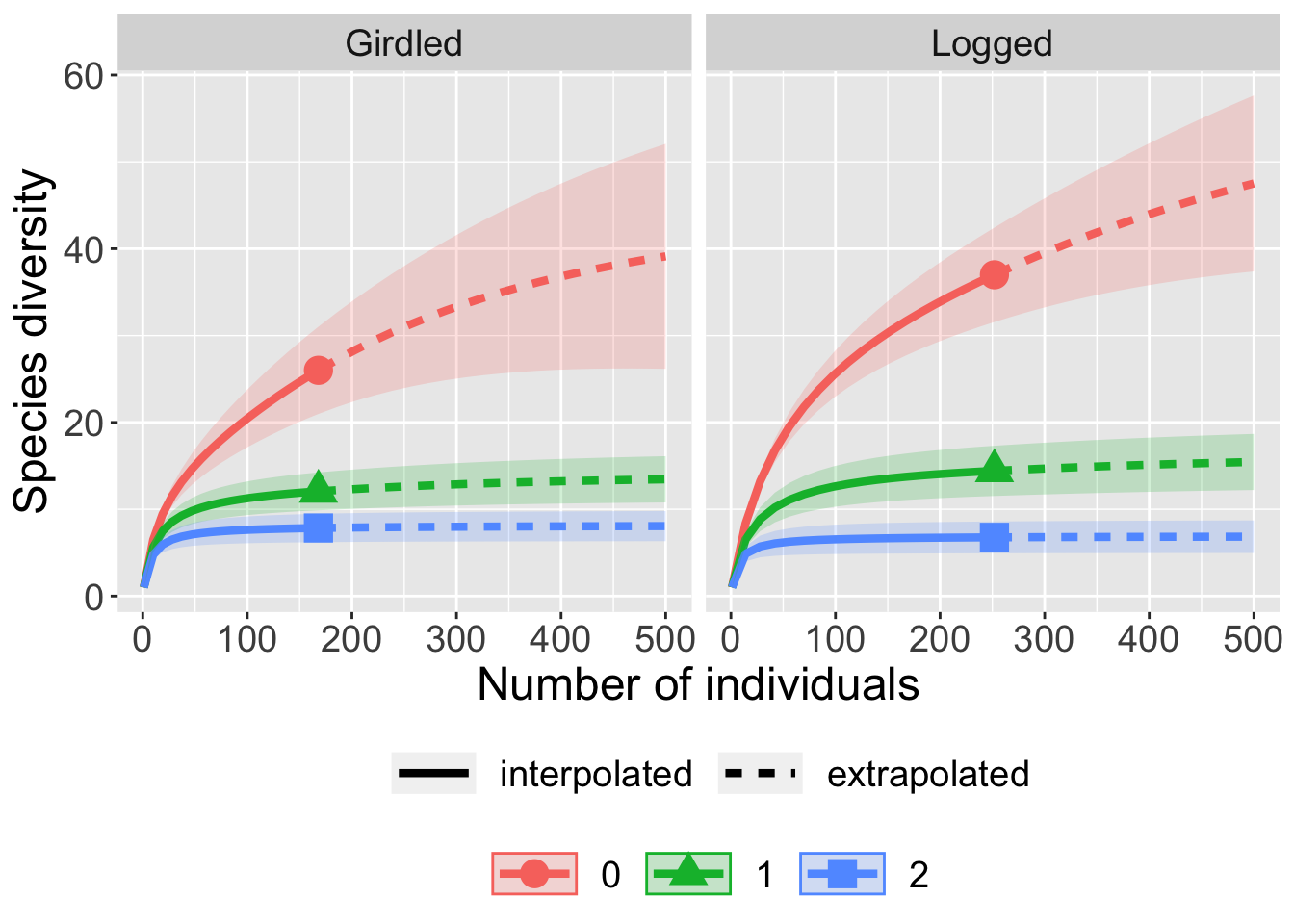The argument facet.var="order" and color.var="site" creates a separate plot for each diversity order site, and within each plot, different colors are used for two sites.

ggiNEXT(out, type=1, facet.var="order", color.var="site")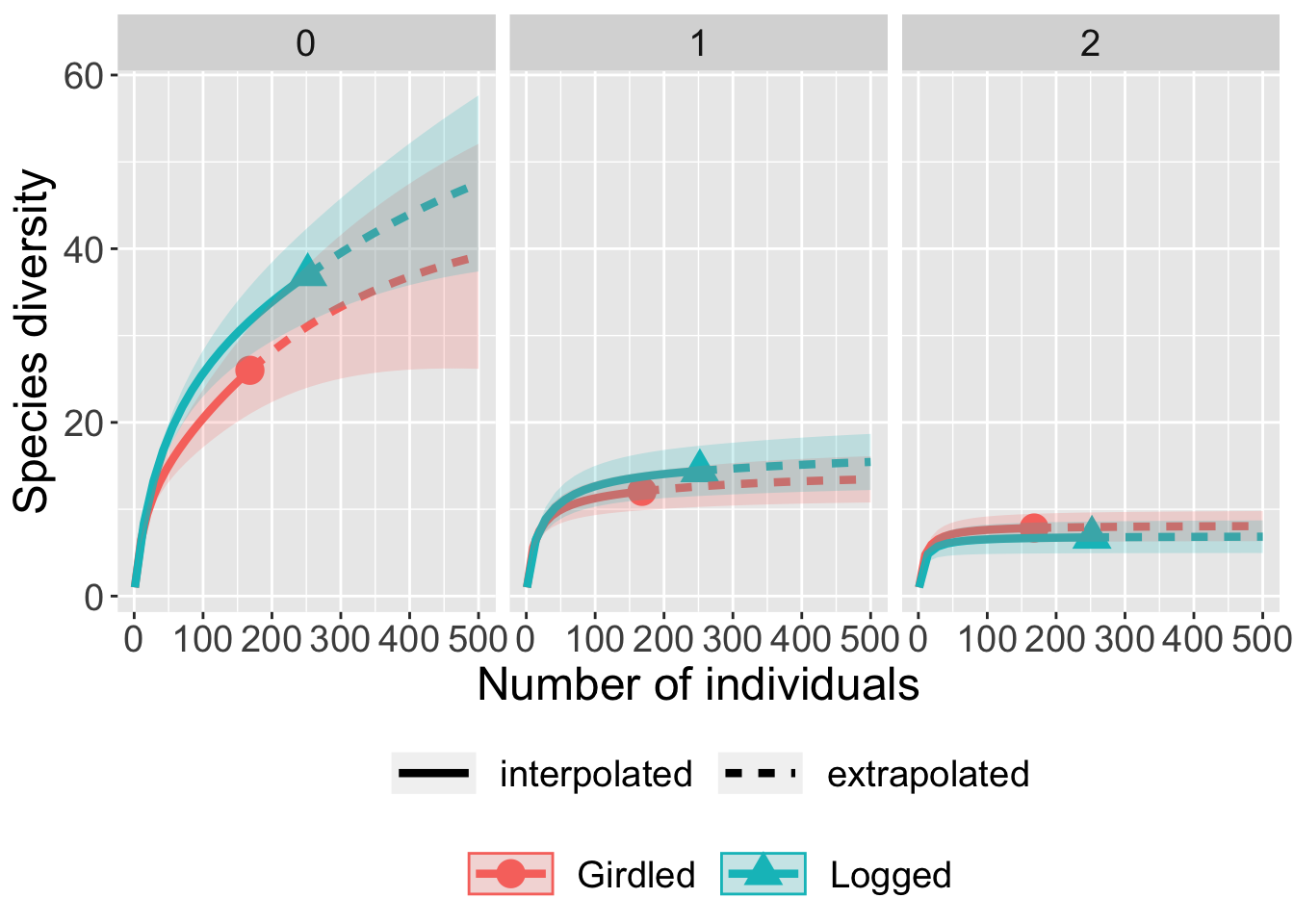The following commands return the sample completeness curve in which different colors are used for the two sites:

ggiNEXT(out, type=2, facet.var="none", color.var="site")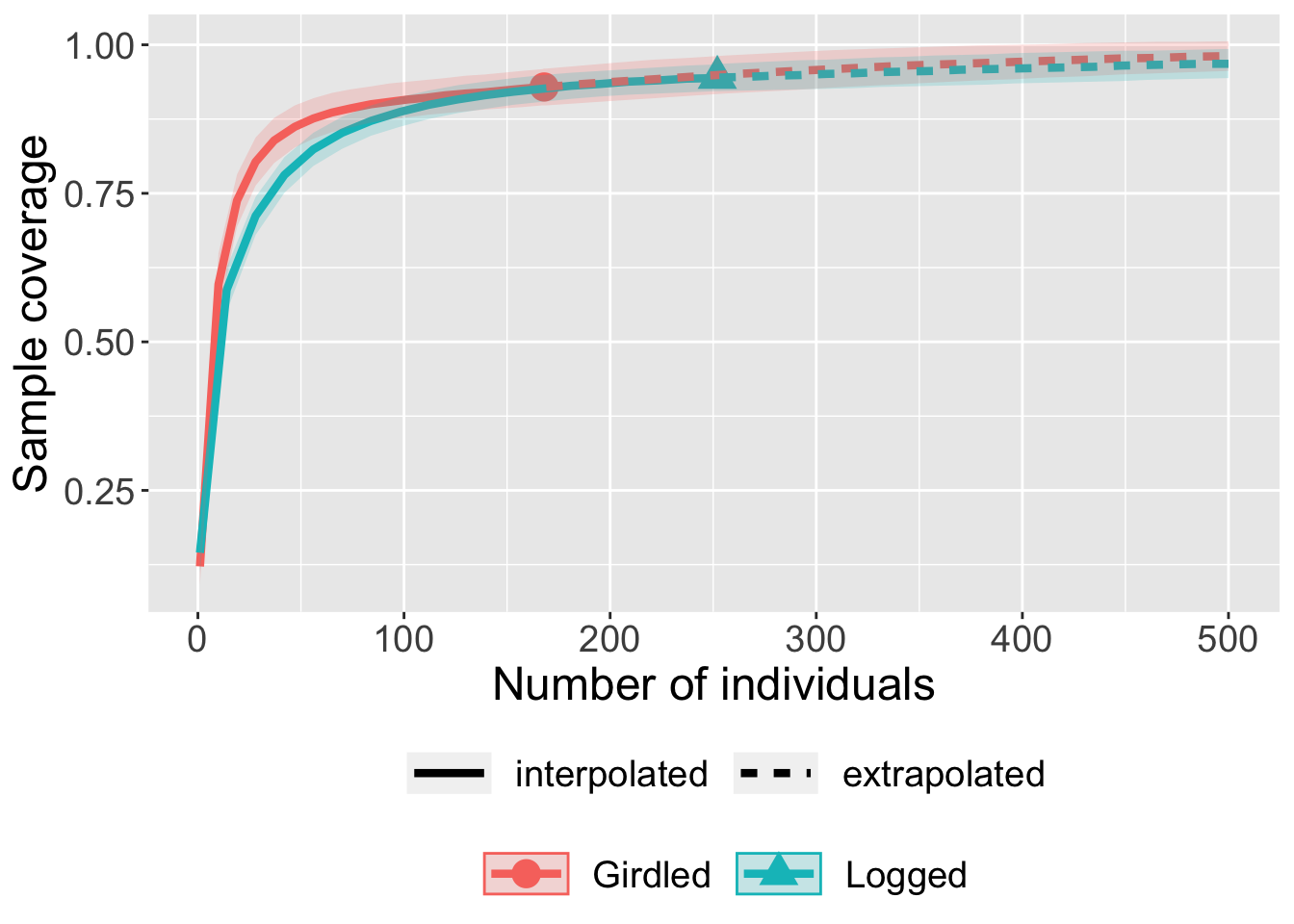The following commands return the coverage‐based R/E sampling curves in which different colors are used for the two sites (facet.var="site") and for three orders (facet.var="order")

ggiNEXT(out, type=3, facet.var="site")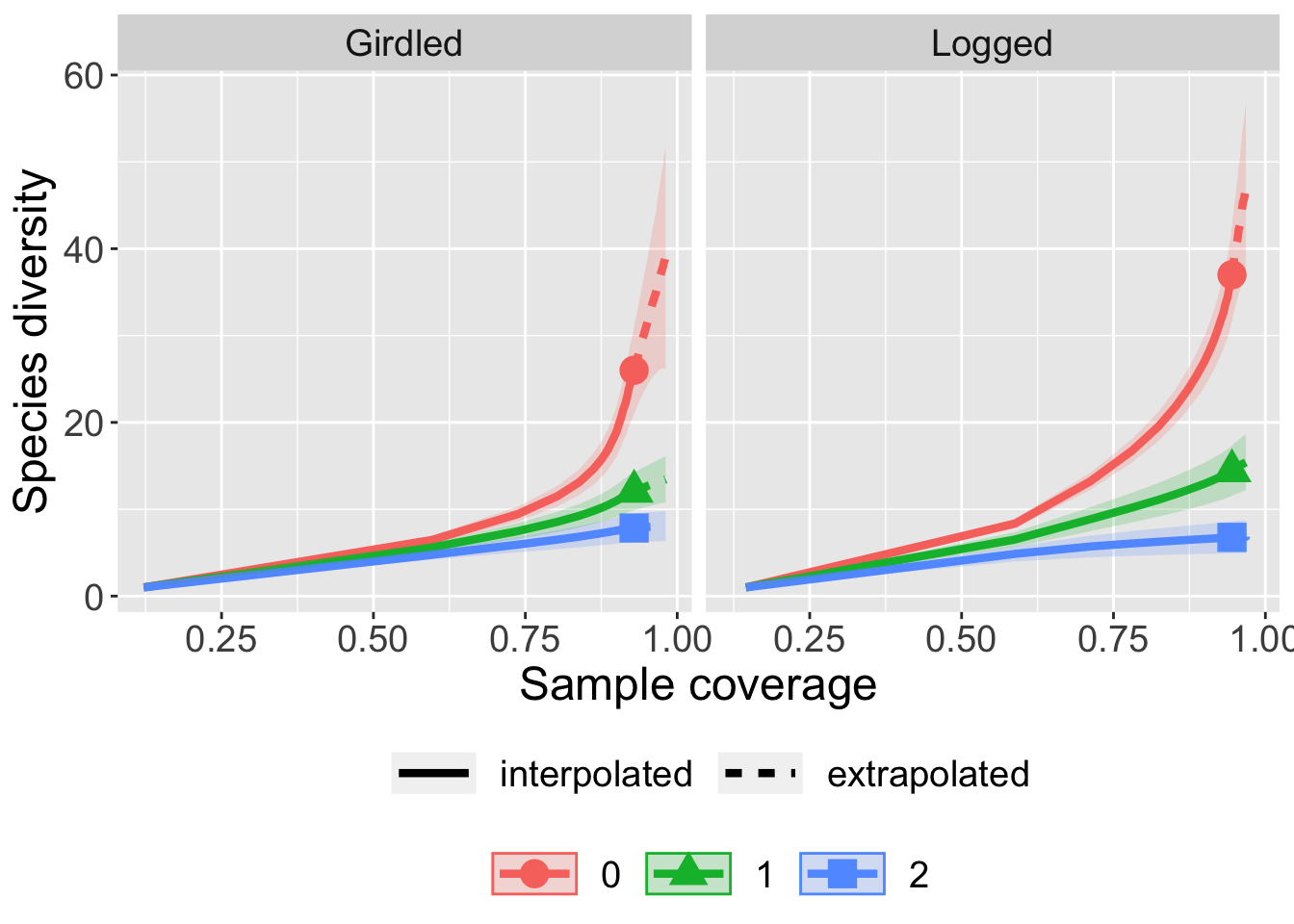ggiNEXT(out, type=3, facet.var="order", color.var="site")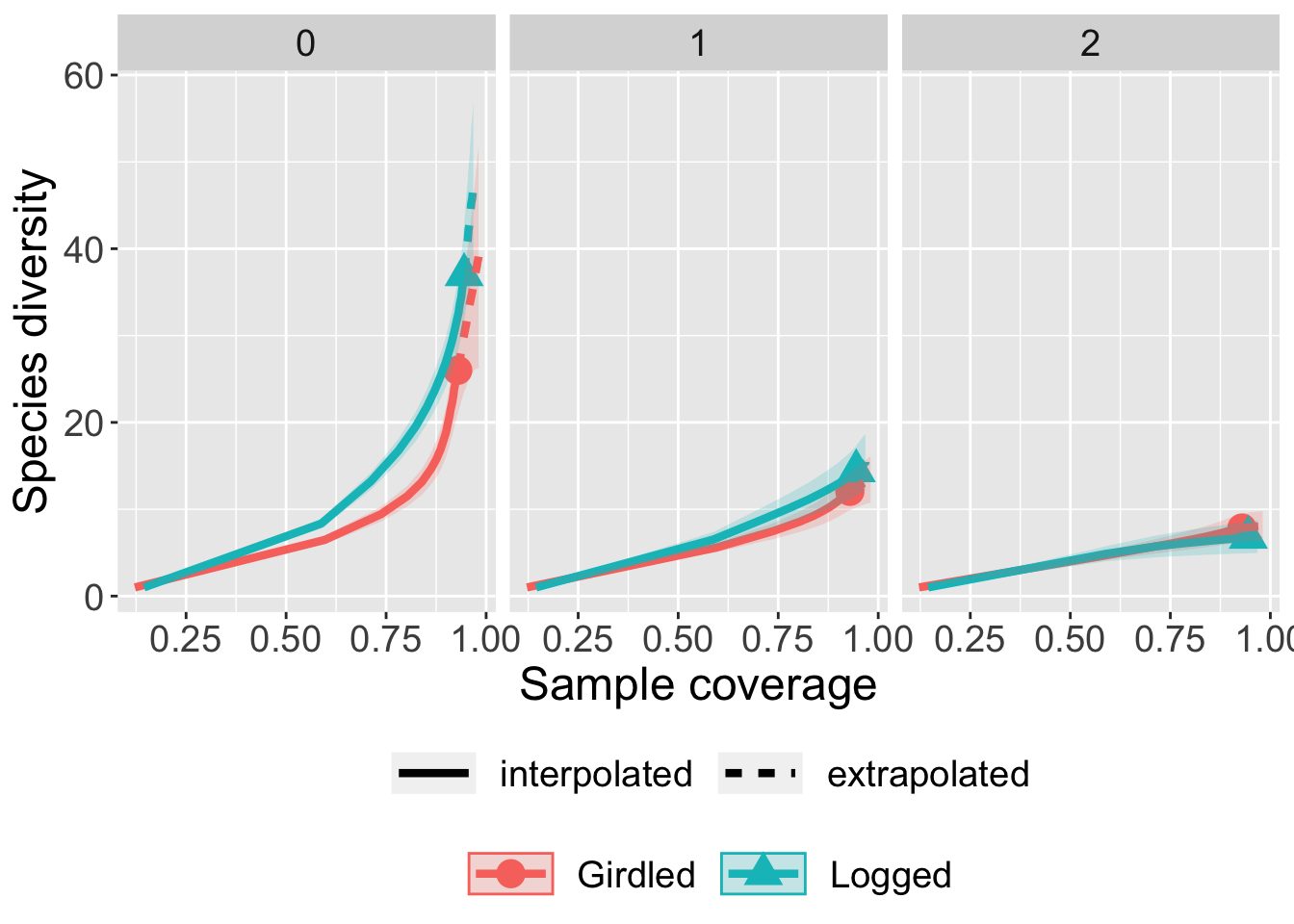## INCIDENCE DATA

For illustration, we use the tropical ant data (in the dataset ant included in the package) at five elevations (50m, 500m, 1070m, 1500m, and 2000m) collected by Longino & Colwell (2011) from Costa Rica. The 5 lists of incidence frequencies are shown below. The first entry of each list must be the total number of sampling units, followed by the species incidence frequencies.

data(ant)
str(ant)
List of 5
$h50m : num [1:228] 599 330 263 236 222 195 186 183 182 129 ...$ h500m : num [1:242] 230 133 131 123 78 73 65 60 60 56 ...
$h1070m: num [1:123] 150 99 96 80 74 68 60 54 46 45 ...$ h1500m: num [1:57] 200 144 113 79 76 74 73 53 50 43 ...
\$ h2000m: num [1:15] 200 80 59 34 23 19 15 13 8 8 ...

The argument color.var = ("none", "order", "site" or "both") is used to display curves in different colors for values of the specified variable. For example, the following code using the argument color.var="site" displays the sampling curves in different colors for the five sites. Note that theme_bw() is a ggplot2 function to modify display setting from grey background to black‐and‐white. The following commands return three types R/E sampling curves for ant data.

t <- seq(1, 700, by=10)
out.inc <- iNEXT(ant, q=0, datatype="incidence_freq", size=t)

# Sample‐size‐based R/E curves
ggiNEXT(out.inc, type=1, color.var="site") +
theme_bw(base_size = 18) +
theme(legend.position="none")